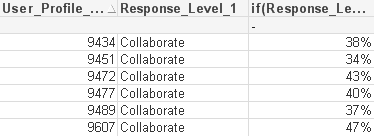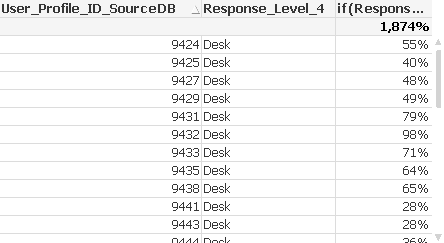# QlikView Creating Analytics

Discussion Board for collaboration related to Creating Analytics for QlikView.Not applicable

## Displaying data in Scatter Chart

Hi Community, stuck on this issue and doesn't seem to be logical - I've create several scatter chart before but can't get the data to display!

I was wondering if it was something to do with the fact that I'm using IF statements?

Any help or direction would be appreciated.

Thanks

Rob

Using the following settings.... (sorry about the size of the expressions)! and all I get is "No Data to Display"

Dimension:

User_Profile_ID_SourceDB

X Axes:

if(Response_Level_1='Collaborate',

if( Sum({\$<Source_Key={1}>}  Ans_Percent)/Sum({\$<Source_Key={1}>} TOTAL<User_Profile_ID_SourceDB> Ans_Percent)

= max(total <User_Profile_ID_SourceDB, Ans_Percent> aggr(Sum({\$<Source_Key={1}>}  Ans_Percent)/Sum({\$<Source_Key={1}>} TOTAL<User_Profile_ID_SourceDB> Ans_Percent), User_Profile_ID_SourceDB, Response_Level_1)),Sum({\$<Source_Key={1}>}  Ans_Percent)/Sum({\$<Source_Key={1}>} TOTAL<User_Profile_ID_SourceDB> Ans_Percent)))

Y Axes:

if(Response_Level_4='Desk',

if( if(Response_Level_2B='Office',(sum ({\$<Source_Key={2},Response_Level_2={'Office'}>} TOTAL<User_Profile_ID_SourceDB> Ans_Percent)/sum ({\$<Source_Key={2}>}TOTAL<User_Profile_ID_SourceDB> Ans_Percent)/1)*sum ({\$<Source_Key={3}, Response_Level_2={'Desk'}>}Ans_Percent)/sum ({\$<Source_Key={3}>}TOTAL<User_Profile_ID_SourceDB> Ans_Percent),if(Source_Key=2,sum ({\$<Source_Key={2}>} Ans_Percent)/sum ({\$<Source_Key={2}>} TOTAL<User_Profile_ID_SourceDB> Ans_Percent),0))

= max(total <User_Profile_ID_SourceDB, Ans_Percent>aggr( if(Response_Level_2B='Office',(sum ({\$<Source_Key={2},Response_Level_2={'Office'}>}TOTAL<User_Profile_ID_SourceDB> Ans_Percent)/sum ({\$<Source_Key={2}>}TOTAL<User_Profile_ID_SourceDB> Ans_Percent)/1)*sum ({\$<Source_Key={3}>}Ans_Percent)/sum ({\$<Source_Key={3}>}TOTAL<User_Profile_ID_SourceDB> Ans_Percent),if(Source_Key=2,sum ({\$<Source_Key={2}>}Ans_Percent)/sum ({\$<Source_Key={2}>} TOTAL<User_Profile_ID_SourceDB> Ans_Percent),0)), User_Profile_ID_SourceDB, Response_Level_4)),

if(Response_Level_2B='Office',(sum ({\$<Source_Key={2},Response_Level_2={'Office'}>}TOTAL<User_Profile_ID_SourceDB> Ans_Percent)/sum ({\$<Source_Key={2}>}TOTAL<User_Profile_ID_SourceDB> Ans_Percent)/1)*sum ({\$<Source_Key={3}>}Ans_Percent)/sum ({\$<Source_Key={3}>}TOTAL<User_Profile_ID_SourceDB> Ans_Percent),if(Source_Key=2,sum ({\$<Source_Key={2}>}Ans_Percent)/sum ({\$<Source_Key={2}>} TOTAL<User_Profile_ID_SourceDB> Ans_Percent),0))))

Tags (1)
4 Replies
HighlightedMVP & Luminary

## Re: Displaying data in Scatter Chart

One possible problem might be caused by the "=" signs in the middle of both expressions, I think this might be a wrong syntax.

Another possible problem is the use of the fields like Response_Level outside of the aggregation functions - if by any chance one of those fields may have multiple available values per  User_Profile_ID_SourceDB, you are likely to get null() values.

What I usually do in those cases is convert the chart to a Straight Table and examine my expressions there.

best,

Oleg Troyansky

www.naturalsynergies.com

HighlightedNot applicable

## Re: Displaying data in Scatter Chart

Thanks Oleg for the suggestions.

Yes this is the frustrating thing - I created two tables to make sure the results I was getting would work before I tried to create the chart.  Both tables create the results I would like to plot of the chart - see below!

Very odd.

You second point might be the issue each of the response levels have either 4 or 5 values per User Profile - however the expression is returning the Max value so reducing it to one result per User Profile.

Any other suggestions you or others might have.

RobHighlightedMVP & Luminary

## Re: Displaying data in Scatter Chart

Rob,

notice that the first table only returns results in the individual rows, but not at the total level. Try removing Response_Level_1 from the list of dimensions and see if you are still getting results.Same with the second table - it looks like you replaced "Expression total" with "Sum of Rows" and therefore you are getting such a high % as a result. Try removing Response_Level_4 from the list of dimensions and see what results you are getting.

Generally speaking, I'd try to find a way to simplify your calculations and to reduce the use of conditions, either using pre-calculated flags or by re-engineering the whole calculation in a different way. It's hard to give you more specific directions without spending the time to understand your business problem and your requirements...

cheers,

Oleg

HighlightedNot applicable

## Re: Displaying data in Scatter Chart

Hi Oleg, thanks for the information.  For the Scatter Chart I had actually removed those dimensions.

Seems to be better when I just tried adding them! the girds appeared.

I will keep working on it, has to be an answer - and appreciate the help.

Thanks

Rob